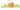# GEL

## Georgian Lari

#### how has the value of the currency changed in the last year?## USD 1 = GEL 2.94

How much is10 US dollars worth inGeorgian laris?
At the current exchange rate, 10 US dollars is worth 29.4 Georgian laris
How much is50 US dollars worth inGeorgian laris?
At the current exchange rate, 50 US dollars is worth 147 Georgian laris
How much is100 US dollars worth inGeorgian laris?
At the current exchange rate, 100 US dollars is worth 294 Georgian laris
How much is500 US dollars worth inGeorgian laris?
At the current exchange rate, 500 US dollars is worth 1,470 Georgian laris
How much is2,000 US dollars worth inGeorgian laris?
At the current exchange rate, 2,000 US dollars is worth 5,880 Georgian laris
How much is10 US dollars worth inGeorgian laris?
At the current exchange rate, 10 US dollars is worth 29.4 Georgian laris
How much is50 US dollars worth inGeorgian laris?
At the current exchange rate, 50 US dollars is worth 147 Georgian laris
How much is100 US dollars worth inGeorgian laris?
At the current exchange rate, 100 US dollars is worth 294 Georgian laris
How much is500 US dollars worth inGeorgian laris?
At the current exchange rate, 500 US dollars is worth 1,470 Georgian laris
How much is2,000 US dollars worth inGeorgian laris?
At the current exchange rate, 2,000 US dollars is worth 5,880 Georgian laris